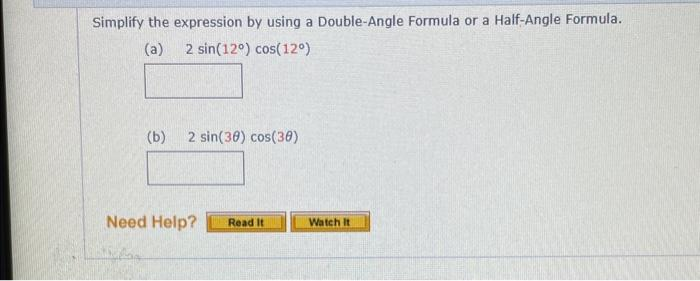# (Solved): help solving  Simplify the expression by using a Double-Angle Formula or a Half-Angle Formula. ...

help solvingSimplify the expression by using a Double-Angle Formula or a Half-Angle Formula. (a) $$2 \sin \left(12^{\circ}\right) \cos \left(12^{\circ}\right)$$ (b) $$2 \sin (3 \theta) \cos (3 \theta)$$

We have an Answer from Expert